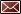Get Email Updates Back to Nonbeverage Products Laboratory page

# Drawback Tutorial

These instructions are for paper submissions; the most efficient way to submit these forms is electronically using Formulas Online. For help on how to register and submit formulas please see our presentation from the Formulas Online Nonbeverage Webinar.

Worksheets for calculating items 9 and 10:

## Simple Mixtures and Filtrations:

Worksheets have been created using 2 sets of units.  Click on the appropriate set of units below to download the calculation worksheet.

pounds / gallons

kilograms / liters

### Simple Mixture 1

Example worksheet.  Formula information on this example can be found here in the tutorial.

### Simple Mix with Yields

Example worksheet.  Formula information on this example can be found here.

* Notice that this formula has a disapproved intermediate, and an actual and theoretical yield.

### 1% Solution Example

Example worksheet.  Formula information on this example can be found here.

* You will see “see tutorial” in the tolerance for box#10.  The value is out of the range the worksheet will calculate.  This occurs with alcohol values ≥ 90% or ≤ 0.5%.  Click here to open the tolerance table from the tutorial and find out the appropriate range for box #10.

## Extracts:

Worksheets have been created using 2 sets of units.  Click on the appropriate set of units below to download the calculation worksheet.

pounds / gallons

kilograms / liters

### Extract 1

Example worksheet. Formula information on this example can be found here in the tutorial.

* The starting menstruum for this is only alcohol and water.

### Extract 2

Example worksheet. Formula information on this example can be found here in the tutorial.

* The starting menstruum for this is alcohol, water, an intermediate and glycerin.

## Dietary Supplements:

Worksheets have been created using 2 sets of units. Click on the appropriate set of units below to download the calculation worksheet.

pounds / gallons

wt. ounces / fluid ounces

### Dietary Supplement 1

Example worksheet. Formula information on this example can be found here.

* This is for a dry herb.

### Dietary Supplement 2

Example worksheet. Formula information on this example can be found here.

* This is for a wet herb with a known moisture content. If there is a range in the moisture content, the average value should be used.